This is “Oxidation and Reduction”, chapter 14 from the book Beginning Chemistry (v. 1.0). For details on it (including licensing), click here.

Has this book helped you? Consider passing it on:
Creative Commons supports free culture from music to education. Their licenses helped make this book available to you.
DonorsChoose.org helps people like you help teachers fund their classroom projects, from art supplies to books to calculators.

# Chapter 14 Oxidation and Reduction

### Opening Essay

Most of us are familiar with rusty iron: metal that has a dark red-brown scale that falls off an object, ultimately weakening it. Although we usually attribute rusting exclusively to iron, this process occurs with many materials. The more formal term for rusting is corrosion.These support beams on a bridge are obviously rusted. If the rusting becomes too bad, it will compromise the integrity of the bridge, requiring replacement.

Corrosion is defined as the disintegration of a material due to chemical reactions with other substances in the environment. In many cases, oxygen in the air causes the disintegration. Corrosion is not uniformly destructive. Although the corrosion of iron is generally considered bad, the corrosion of aluminum and copper forms a protective barrier on the surface of the metal, protecting it from further reaction with the environment.

Having said that, it has been estimated that as much as 5% of expenditures in the United States apply to fixing problems caused by corrosion. The replacement of structures built with iron, steel, aluminum, and concrete must be performed regularly to keep these structures safe. As an example of what might happen, consider the story of the Silver Bridge on US Interstate 35, connecting West Virginia and Ohio. On December 15, 1967, the 39-year-old bridge collapsed, killing 46 people. The ultimate cause of the collapse was determined to be corrosion of a suspension chain on the Ohio side of the bridge.

Corrosion is an example of the type of chemical reaction discussed in this chapter. Although we usually think of corrosion as bad, the reaction it typifies can actually be put to good use.

One important type of chemical reaction is the oxidation-reduction reaction, also known as the redox reaction. Although we introduced redox reactions in Chapter 4 "Chemical Reactions and Equations", Section 4.6 "Oxidation-Reduction Reactions", it is worth reviewing some basic concepts.

## 14.1 Oxidation-Reduction Reactions

### Learning Objectives

1. Define oxidation and reduction.
2. Assign oxidation numbers to atoms in simple compounds.
3. Recognize a reaction as an oxidation-reduction reaction.

Consider this chemical reaction:

Mg(s) + Cl2(g) → MgCl2

The reactants are two electrically neutral elements; they have the same number of electrons as protons. The product, however, is ionic; it is composed of Mg2+ and Cl ions. Somehow, the individual Mg atoms lose two electrons to make the Mg2+ ion, while the Cl atoms gain an electron to become Cl ions. This reaction involves the transfer of electrons between atoms.

The process of losing and gaining electrons occurs simultaneously. However, mentally we can separate the two processes. OxidationThe loss of one or more electrons by an atom; an increase in oxidation number. is defined as the loss of one or more electrons by an atom. ReductionThe gain of one or more electrons by an atom; a decrease in oxidation number. is defined as the gain of one or more electrons by an atom. So oxidation and reduction always occur together; it is only mentally that we can separate them. Chemical reactions that involve the transfer of electrons are called oxidation-reduction (or redox) reactionsA chemical reaction that involves the transfer of electrons..

Redox reactions require that we keep track of the electrons assigned to each atom in a chemical reaction. How do we do that? We use oxidation numbersA number assigned to an atom that helps keep track of the number of electrons on the atom. to keep track of electrons in atoms. Oxidation numbers are assigned to atoms based on four rules. Oxidation numbers are not necessarily equal to the charge on the atom (although sometimes they can be); we must keep the concepts of charge and oxidation numbers separate.

The rules for assigning oxidation numbers to atoms are as follows:

1. Atoms in their elemental state are assigned an oxidation number of 0.
2. Atoms in monatomic (i.e., one-atom) ions are assigned an oxidation number equal to their charge. Oxidation numbers are usually written with the sign first, then the magnitude, to differentiate them from charges.
3. In compounds, fluorine is assigned a −1 oxidation number; oxygen is usually assigned a −2 oxidation number [except in peroxide compounds (where it is −1) and in binary compounds with fluorine (where it is positive)]; and hydrogen is usually assigned a +1 oxidation number [except when it exists as the hydride ion (H), in which case rule 2 prevails].
4. In compounds, all other atoms are assigned an oxidation number so that the sum of the oxidation numbers on all the atoms in the species equals the charge on the species (which is zero if the species is neutral).

Here are some examples for practice. In H2, both H atoms have an oxidation number of 0 by rule 1. In MgCl2, magnesium has an oxidation number of +2, while chlorine has an oxidation number of −1 by rule 2. In H2O, the H atoms each have an oxidation number of +1, while the O atom has an oxidation number of −2, even though hydrogen and oxygen do not exist as ions in this compound (rule 3). By contrast, by rule 3, each H atom in hydrogen peroxide (H2O2) has an oxidation number of +1, while each O atom has an oxidation number of −1. We can use rule 4 to determine oxidation numbers for the atoms in SO2. Each O atom has an oxidation number of −2; for the sum of the oxidation numbers to equal the charge on the species (which is zero), the S atom is assigned an oxidation number of +4. Does this mean that the sulfur atom has a 4+ charge on it? No, it means only that the S atom is assigned a +4 oxidation number by our rules of apportioning electrons among the atoms in a compound.

### Example 1

Assign oxidation numbers to the atoms in each substance.

1. Cl2
2. GeO2
3. Ca(NO3)2

Solution

1. Cl2 is the elemental form of chlorine. Rule 1 states each atom has an oxidation number of 0.
2. By rule 3, oxygen is normally assigned an oxidation number of −2. For the sum of the oxidation numbers to equal the charge on the species (zero), the Ge atom is assigned an oxidation number of +4.
3. Ca(NO3)2 can be separated into two parts: the Ca2+ ion and the NO3 ion. Considering these separately, the Ca2+ ion has an oxidation number of +2 by rule 2. Now consider the NO3 ion. Oxygen is assigned an oxidation number of −2, and there are three of them. According to rule 4, the sum of the oxidation numbers on all atoms must equal the charge on the species, so we have the simple algebraic equation

x + 3(−2) = −1

where x is the oxidation number of the N atom and the −1 represents the charge on the species. Evaluating for x,

x + (−6) = −1 x = +5

Thus the oxidation number on the N atom in the NO3 ion is +5.

Test Yourself

Assign oxidation numbers to the atoms in H3PO4.

H: +1; O: −2; P: +5

All redox reactions occur with a simultaneous change in the oxidation numbers of some atoms. At least two elements must change their oxidation numbers. When an oxidation number of an atom is increased in the course of a redox reaction, that atom is being oxidized. When an oxidation number of an atom is decreased in the course of a redox reaction, that atom is being reduced. Thus oxidation and reduction can also be defined in terms of increasing or decreasing oxidation numbers, respectively.

### Example 2

Identify what is being oxidized and reduced in this redox reaction.

2Na + Br2 → 2NaBr

Solution

Both reactants are the elemental forms of their atoms, so the Na and Br atoms have oxidation numbers of 0. In the ionic product, the Na+ ions have an oxidation number of +1, while the Br ions have an oxidation number of −1.

Sodium is increasing its oxidation number from 0 to +1, so it is being oxidized; bromine is decreasing its oxidation number from 0 to −1, so it is being reduced: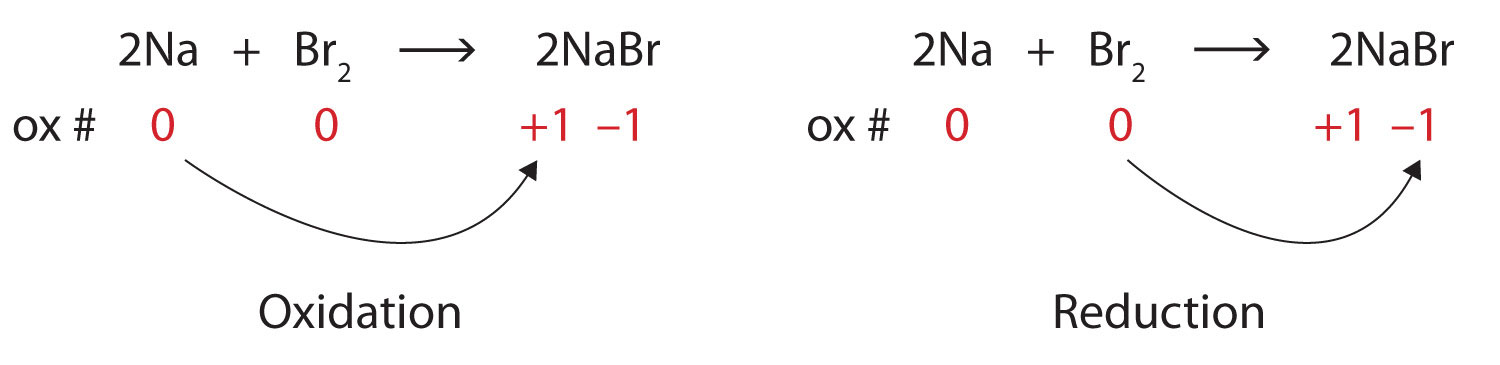Because oxidation numbers are changing, this is a redox reaction. The total number of electrons being lost by sodium (two, one lost from each Na atom) is gained by bromine (two, one gained for each Br atom).

Test Yourself

Identify what is being oxidized and reduced in this redox reaction.

C + O2 → CO2

C is being oxidized from 0 to +4; O is being reduced from 0 to −2.

Oxidation reactions can become quite complex, as attested by the following redox reaction:

$6H+(aq)+2Mn+7O4−(aq)+5H2O2−1(ℓ) →2Mn2++2(aq)+5O20(g)+8H2O(ℓ)$

To demonstrate that this is a redox reaction, the oxidation numbers of the species being oxidized and reduced are listed; can you determine what is being oxidized and what is being reduced? This is also an example of a net ionic reaction; spectator ions that do not change oxidation numbers are not displayed in the equation. Eventually, we will need to learn techniques for writing correct (i.e., balanced) redox reactions.

### Food and Drink App: Fortifying Food with Iron

Iron is an essential mineral in our diet; iron-containing compounds like the heme protein in hemoglobin could not function without it. Most biological iron has the form of the Fe2+ ion; iron with other oxidation numbers is almost inconsequential in human biology (although the body does contain an enzyme to reduce Fe3+ to Fe2+, so Fe3+ must have some biological significance, albeit minor). To ensure that we ingest enough iron, many foods are enriched with iron. Although Fe2+ compounds are the most logical substances to use, some foods—bread and breakfast cereals are the most well-known examples—use “reduced iron” as an ingredient. Reduced iron is simply iron metal; iron is added as a fine metallic powder. The metallic iron is oxidized to Fe2+ in the digestive system and then absorbed by the body, but the question remains: Why are we ingesting metallic iron? Why not just use Fe2+ salts as an additive?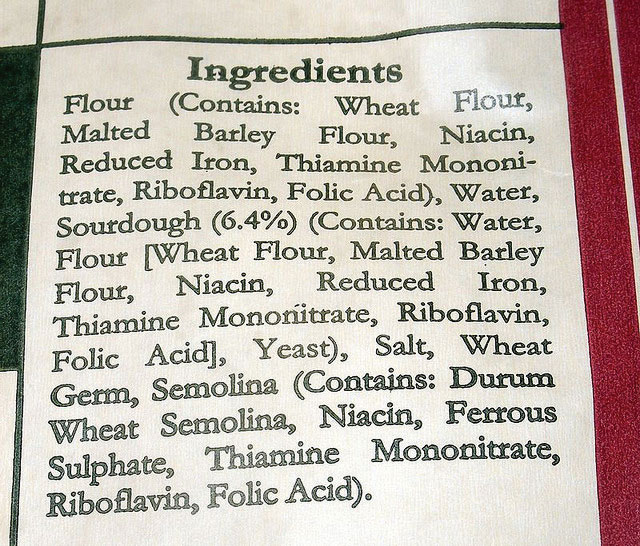Many prepared foods list reduced iron in their ingredients list.

Although it is difficult to establish conclusive reasons, a search of scientific and medical literature suggests a few reasons. One reason is that fine iron filings do not affect the taste of the product. The size of the iron powder (several dozen micrometers) is not noticeable when chewing iron-supplemented foods, and the tongue does not detect any changes in flavor that can be detected when using Fe2+ salts. Fe2+ compounds can affect other properties of foodstuffs during preparation and cooking, like dough pliability, yeast growth, and color. Finally, of the common iron substances that might be used, metallic iron is the least expensive. These factors appear to be among the reasons why metallic iron is the supplement of choice in some foods.

### Key Takeaways

• Oxidation-reduction (redox) reactions involve the transfer of electrons from one atom to another.
• Oxidation numbers are used to keep track of electrons in atoms.
• There are rules for assigning oxidation numbers to atoms.
• Oxidation is an increase in oxidation number (loss of electrons); reduction is a decrease in oxidation number (gain of electrons).

### Exercises

1. Is this reaction a redox reaction? Explain your answer.

2K(s) + Br2(ℓ) → 2KBr(s)
2. Is this reaction a redox reaction? Explain your answer.

2NaCl(aq) + Pb(NO3)2(aq) → 2NaNO3(aq) + PbCl2(s)
3. Which substance loses electrons and which substance gains electrons in this reaction?

2Mg(s) + O2(g) → 2MgO
4. Which substance loses electrons and which substance gains electrons in this reaction?

16Fe(s) + 3S8(s) → 8Fe2S3(s)
5. Which substance is oxidized and which substance is reduced in this reaction?

2Li(s) + O2(g) → Li2O2(s)
6. Which substance is oxidized and which substance is reduced in this reaction?

2Fe(s) + 3I2(s) → 2FeI3(s)
7. What are two different definitions of oxidation?

8. What are two different definitions of reduction?

9. Assign oxidation numbers to the atoms in each substance.

1. P4
2. SO3
3. SO32−
4. Ca3(PO3)2
10. Assign oxidation numbers to the atoms in each substance.

1. PCl5
2. (NH4)2Se
3. Ag
4. Li2O2
11. Assign oxidation numbers to the atoms in each substance.

1. NO
2. NO2
3. CrCl2
4. CrCl3
12. Assign oxidation numbers to the atoms in each substance.

1. NaH
2. N2O3
3. NO2
4. CuNO3
13. Assign oxidation numbers to the atoms in each substance.

1. CH2O
2. NH3
3. Rb2SO4
4. Zn(C2H3O2)2
14. Assign oxidation numbers to the atoms in each substance.

1. C6H6
2. B(OH)3
3. Li2S
4. Au
15. Identify what is being oxidized and reduced in this redox reaction by assigning oxidation numbers to the atoms.

2NO + Cl2 → 2NOCl
16. Identify what is being oxidized and reduced in this redox reaction by assigning oxidation numbers to the atoms.

Sr + SO3 → SrSO3
17. Identify what is being oxidized and reduced in this redox reaction by assigning oxidation numbers to the atoms.

2KrF2 + 2H2O → 2Kr + 4HF + O2
18. Identify what is being oxidized and reduced in this redox reaction by assigning oxidation numbers to the atoms.

SO3 + SCl2 → SOCl2 + SO2
19. Identify what is being oxidized and reduced in this redox reaction by assigning oxidation numbers to the atoms.

2Rb + MgCl2 → 2RbCl + Mg
20. Identify what is being oxidized and reduced in this redox reaction by assigning oxidation numbers to the atoms.

2C8H18 + 25O2 → 16CO2 + 18H2O

1. yes because oxidation numbers are changing

2. lose: Mg; gain: O

3. oxidized: Li; reduced: O

4. increase in oxidation number; loss of electrons

1. P: 0
2. S: +6; O: −2
3. S: +4; O: −2
4. Ca: +2; P: +3; O: −2
1. N: +2; O: −2
2. N: +4; O: −2
3. Cr: +2; Cl: −1
4. Cr: +3; Cl: −1
1. C: 0; H: +1; O: −2
2. N: −3; H: +1
3. Rb: +1; S: +6; O: −2
4. Zn: +2; C: 0; H: +1; O: −2
5. oxidized: N; reduced: Cl

6. oxidized: O; reduced: Kr

7. oxidized: Rb; reduced: Mg

## 14.2 Balancing Redox Reactions

### Learning Objectives

1. Learn to balanced simple redox reactions by inspection.
2. Learn to balance complex redox reactions by the half reaction method.
3. Use the solvent, or parts of it, as a reactant or a product in balancing a redox reaction.

Balancing simple redox reactions can be a straightforward matter of going back and forth between products and reactants. For example, in the redox reaction of Na and Cl2:

Na + Cl2 → NaCl

it should be immediately clear that the Cl atoms are not balanced. We can fix this by putting the coefficient 2 in front of the product:

Na + Cl2 → 2NaCl

However, now the sodium is unbalanced. This can be fixed by including the coefficient 2 in front of the Na reactant:

2Na + Cl2 → 2NaCl

This reaction is now balanced. That was fairly straightforward; we say that we are able to balance the reaction by inspection. Many simple redox reactions can be balanced by inspection.

### Example 3

Balance this redox reaction by inspection.

SO2 + O2 → SO3

Solution

There is one S atom on both sides of the equation, so the sulfur is balanced. However, the reactant side has four O atoms while the product side has three. Clearly we need more O atoms on the product side, so let us start by including the coefficient 2 on the SO3:

SO2 + O2 → 2SO3

This now gives us six O atoms on the product side, and it also imbalances the S atoms. We can balance both the elements by adding coefficient 2 on the SO2 on the reactant side:

2SO2 + O2 → 2SO3

This gives us two S atoms on both sides and a total of six O atoms on both sides of the chemical equation. This redox reaction is now balanced.

Test Yourself

Balance this redox reaction by inspection.

Al + O2 → Al2O3

4Al + 3O2 → 2Al2O3

The first thing you should do when encountering an unbalanced redox reaction is to try to balance it by inspection.

Some redox reactions are not easily balanced by inspection. Consider this redox reaction:

Al + Ag+ → Al3+ + Ag

At first glance, this equation seems balanced: there is one Ag atom on both sides and one Al atom on both sides. However, if you look at the total charge on each side, there is a charge imbalance: the reactant side has a total charge of 1+, while the product side has a total charge of 3+. Something is amiss with this chemical equation; despite the equal number of atoms on each side, it is not balanced.

A fundamental point about redox reactions that has not arisen previously is that the total number of electrons being lost must equal the total number of electrons being gained for a redox reaction to be balanced. This is not the case for the aluminum and silver reaction: the Al atom loses three electrons to become the Al3+ ion, while the Ag+ ion gains only one electron to become elemental silver.

To balance this, we will write each oxidation and reduction reaction separately, listing the number of electrons explicitly in each. Individually, the oxidation and reduction reactions are called half reactionsThe individual oxidation or reduction reaction of a redox reaction.. We will then take multiples of each reaction until the number of electrons on each side cancels completely and combine the half reactions into an overall reaction, which should then be balanced. This method of balancing redox reactions is called the half reaction methodThe method of balancing redox reactions by writing and balancing the individual half reactions.. (There are other ways of balancing redox reactions, but this is the only one that will be used in this text. The reason for this will be seen in Chapter 14 "Oxidation and Reduction", Section 14.3 "Applications of Redox Reactions: Voltaic Cells".)

The oxidation half reaction involves aluminum, which is being oxidized:

Al → Al3+

This half reaction is not completely balanced because the overall charges on each side are not equal. When an Al atom is oxidized to Al3+, it loses three electrons. We can write these electrons explicitly as products:

Al → Al3+ + 3e

Now this half reaction is balanced—in terms of both atoms and charges.

The reduction half reaction involves silver:

Ag+ → Ag

The overall charge is not balanced on both sides. But we can fix this by adding one electron to the reactant side because the Ag+ ion must accept one electron to become the neutral Ag atom:

Ag+ + e → Ag

This half reaction is now also balanced.

When combining the two half reactions into a balanced chemical equation, the key is that the total number of electrons must cancel, so the number of electrons lost by atoms are equal to the number of electrons gained by other atoms. This may require we multiply one or both half reaction(s) by an integer to make the number of electrons on each side equal. With three electrons as products and one as reactant, the least common multiple of these two numbers is three: we can use a single aluminum reaction but must take three times the silver reaction:

Al → Al3+ + 3e 3 × [Ag+ + e → Ag]

The 3 on the second reaction is distributed to all species in the reaction:

Al → Al3+ + 3e 3Ag+ + 3e → 3Ag

Now the two half reactions can be combined just like two algebraic equations, with the arrow serving as the equals sign. The same species on opposite sides of the arrow can be canceled:

The net balanced redox reaction is as follows:

Al + 3Ag+ → Al3+ + 3Ag

There is still only one Al atom on each side of the chemical equation, but there are now three Ag atoms, and the total charge on each side of the equation is the same (3+ for both sides). This redox reaction is balanced. It took more effort to use the half reaction method than by inspection, but the correct balanced redox reaction was obtained.

### Example 4

Balance this redox reaction by using the half reaction method.

Fe2+ + Cr → Fe + Cr3+

Solution

We start by writing the two half reactions. Chromium is being oxidized, and iron is being reduced:

Cr → Cr3+ oxidation Fe2+ → Fe reduction

Then we include the appropriate number of electrons on the proper side to balance the charges for each reaction:

Cr → Cr3+ + 3e Fe2+ + 2e → Fe

The first reaction involves three electrons, while the second reaction involves two electrons. The least common multiple of these two numbers is six, so to get six electrons in each reaction we need to double the first reaction and triple the second one:

2 × [Cr → Cr3+ + 3e] = 2Cr → 2Cr3+ + 6e 3 × [Fe2+ + 2e → Fe] = 3Fe2+ + 6e → 3Fe

We can combine the two final reactions, noting that the electrons cancel:

The overall, balanced redox reaction is

2Cr + 3Fe2+ → 2Cr3+ + 3Fe

Test Yourself

Balance this redox reaction by using the half reaction method.

O2− + F2 → O2 + F

2O2− + 2F2 → O2 + 4F

Many redox reactions occur in aqueous solution—in water. Because of this, in many cases H2O or a fragment of an H2O molecule (H+ or OH, in particular) can participate in the redox reaction. As such, we need to learn how to incorporate the solvent into a balanced redox equation.

Consider the following oxidation half reaction in aqueous solution, which has one Cr atom on each side:

Cr3+ → CrO4

Here, the Cr atom is going from the +3 to the +7 oxidation state. To do this, the Cr atom must lose four electrons. Let us start by listing the four electrons as products:

Cr3+ → CrO4 + 4e

But where do the O atoms come from? They come from water molecules or a common fragment of a water molecule that contains an O atom: the OH ion. When we balance this half reaction, we should feel free to include either of these species in the reaction to balance the elements. Let us use H2O to balance the O atoms; we need to include four water molecules to balance the four O atoms in the products:

4H2O + Cr3+ → CrO4 + 4e

This balances the O atoms, but now introduces hydrogen to the reaction. We can balance the H atoms by adding an H+ ion, which is another fragment of the water molecule. We need to add eight H+ ions to the product side:

4H2O + Cr3+ → CrO4 + 4e + 8H+

The Cr atoms are balanced, the O atoms are balanced, and the H atoms are balanced; if we check the total charge on both sides of the chemical equation, they are the same (3+, in this case). This half reaction is now balanced, using water molecules and parts of water molecules as reactants and products.

Reduction reactions can be balanced in a similar fashion. When oxidation and reduction half reactions are individually balanced, they can be combined in the same fashion as before: by taking multiples of each half reaction as necessary to cancel all electrons. Other species, such as H+, OH, and H2O, may also have to be canceled in the final balanced reaction.

Unless otherwise noted, it does not matter if you add H2O or OH as a source of O atoms, although a reaction may specify acidic solution or basic solution as a hint of what species to use or what species to avoid. OH ions are not very common in acidic solutions, so they should be avoided in those circumstances.

### Example 5

Balance this redox reaction. Assume a basic solution.

MnO2 + CrO3 → Mn + CrO4

Solution

We start by separating the oxidation and reduction processes so we can balance each half reaction separately. The oxidation reaction is as follows:

CrO3 → CrO4

The Cr atom is going from a +5 to a +7 oxidation state and loses two electrons in the process. We add those two electrons to the product side:

CrO3 → CrO4 + 2e

Now we must balance the O atoms. Because the solution is basic, we should use OH rather than H2O:

OH + CrO3 → CrO4 + 2e

We have introduced H atoms as part of the reactants; we can balance them by adding H+ as products:

OH + CrO3 → CrO4 + 2e + H+

If we check the atoms and the overall charge on both sides, we see that this reaction is balanced. However, if the reaction is occurring in a basic solution, it is unlikely that H+ ions will be present in quantity. The way to address this is to add an additional OH ion to each side of the equation:

OH + CrO3 + OH → CrO4 + 2e + H+ + OH

The two OH ions on the left side can be grouped together as 2OH. On the right side, the H+ and OH ions can be grouped into an H2O molecule:

2OH + CrO3 → CrO4 + 2e + H2O

This is a more appropriate form for a basic solution.

Now we balance the reduction reaction:

MnO2 → Mn

The Mn atom is going from +4 to 0 in oxidation number, which requires a gain of four electrons:

4e + MnO2 → Mn

Then we balance the O atoms and then the H atoms:

4e + MnO2 → Mn + 2OH 2H+ + 4e + MnO2 → Mn + 2OH

We add two OH ions to each side to eliminate the H+ ion in the reactants; the reactant species combine to make two water molecules, and the number of OH ions in the product increases to four:

2H2O + 4e + MnO2 → Mn + 4OH

This reaction is balanced for a basic solution.

Now we combine the two balanced half reactions. The oxidation reaction has two electrons, while the reduction reaction has four. The least common multiple of these two numbers is four, so we multiply the oxidation reaction by 2 so that the electrons are balanced:

2 × [2OH + CrO3 → CrO4 + 2e + H2O] 2H2O + 4e + MnO2 → Mn + 4OH

Combining these two equations results in the following equation:

4OH + 2CrO3 + 2H2O + 4e + MnO2 → 2CrO4 + 4e + 2H2O + Mn + 4OH

The four electrons cancel. So do the two H2O molecules and the four OH ions. What remains is

2CrO3 + MnO2 → 2CrO4 + Mn

which is our final balanced redox reaction.

Test Yourself

Balance this redox reaction. Assume a basic solution.

Cl + MnO4 → MnO2 + ClO3

H2O + Cl + 2MnO4 → 2MnO2 + ClO3 + 2OH

### Key Takeaways

• Redox reactions can be balanced by inspection or by the half reaction method.
• A solvent may participate in redox reactions; in aqueous solutions, H2O, H+, and OH may be reactants or products.

### Exercises

1. Balance these redox reactions by inspection.

1. Na + F2 → NaF
2. Al2O3 + H2 → Al + H2O
2. Balance these redox reactions by inspection.

1. Fe2S3 + O2 → Fe2O3 + S
2. Cu2O + H2 → Cu + H2O
3. Balance these redox reactions by inspection.

1. CH4 + O2 → CO2 + H2O
2. P2O5 + Cl2 → PCl3 + O2
4. Balance these redox reactions by inspection.

1. PbCl2 + FeCl3 → PbCl4 + FeCl2
2. SO2 + F2 → SF4 + OF2
5. Balance these redox reactions by the half reaction method.

1. Ca + H+ → Ca2+ + H2
2. Sn2+ → Sn + Sn4+ (Hint: both half reactions will start with the same reactant.)
6. Balance these redox reactions by the half reaction method.

1. Fe3+ + Sn2+ → Fe + Sn4+
2. Pb2+ → Pb + Pb4+ (Hint: both half reactions will start with the same reactant.)
7. Balance these redox reactions by the half reaction method.

1. Na + Hg2Cl2 → NaCl + Hg
2. Al2O3 + C → Al + CO2
8. Balance these redox reactions by the half reaction method.

1. Br + I2 → I + Br2
2. CrCl3 + F2 → CrF3 + Cl2
9. Balance these redox reactions that occur in aqueous solution. Use whatever water-derived species is necessary; there may be more than one correct balanced equation.

1. Cu + NO3 → Cu2+ + NO2
2. Fe + MnO4 → Fe3+ + Mn
10. Balance these redox reactions that occur in aqueous solution. Use whatever water-derived species is necessary; there may be more than one correct balanced equation.

1. CrO3 + Ni2+ → Cr3+ + Ni3+
2. OsO4 + C2H4 → Os + CO2
11. Balance these redox reactions that occur in aqueous solution. Use whatever water-derived species is necessary; there may be more than one correct balanced equation.

1. ClO + Ti2+ → Ti4+ + Cl
2. BrO3 + Ag → Ag+ + BrO2
12. Balance these redox reactions that occur in aqueous solution. Use whatever water-derived species is necessary; there may be more than one correct balanced equation.

1. H2O2 + NO → N2O3 + H2O
2. VO2+ + NO → V3+ + NO2
13. Explain why this chemical equation is not balanced and balance it if it can be balanced.

Cr2+ + Cl2 → Cr3+ + 2Cl
14. Explain why this equation is not balanced and balance it if it can be balanced.

O2 + 2H2O + Br2 → 4OH + 2Br

1. 2Na + F2 → 2NaF
2. Al2O3 + 3H2 → 2Al + 3H2O
1. CH4 + 2O2 → CO2 + 2H2O
2. 2P2O5 + 6Cl2 → 4PCl3 + 5O2
1. Ca + 2H+ → Ca2+ + H2
2. 2Sn2+ → Sn + Sn4+
1. 2Na + Hg2Cl2 → 2NaCl + 2Hg
2. 2Al2O3 + 3C → 4Al + 3CO2
1. 4H+ + Cu + 2NO3 → Cu2+ + 2NO2 + 2H2O in acidic solution; 2H2O + Cu + 2NO3 → Cu2+ + 2NO2 + 4OH in basic solution
2. 24H+ + 3MnO4 + 7Fe → 7Fe3+ + 3Mn + 12H2O in acidic solution; 12H2O + 3MnO4 + 7Fe → 7Fe3+ + 3Mn + 24OH in basic solution
1. 2H+ + ClO + Ti2+ → Cl + H2O + Ti4+ in acidic solution; H2O + ClO + Ti2+ → Cl + Ti4+ + 2OH in basic solution
2. 2H+ + BrO3 + Ag → BrO2 + H2O + Ag+ in acidic solution; H2O + BrO3 + Ag → BrO2 + Ag+ + 2OH in basic solution
1. The charges are not properly balanced. The correct balanced equation is 2Cr2+ + Cl2 → 2Cr3+ + 2Cl.

## 14.3 Applications of Redox Reactions: Voltaic Cells

### Learning Objectives

1. Learn the parts of a voltaic cell.
2. Combine half reactions to determine the voltage of a voltaic cell.
3. Understand how voltaic cells are used as batteries.

Consider this redox reaction:

Zn + Cu2+ → Zn2+ + Cu

If you were to mix zinc metal and copper ions in a container, this reaction would proceed by itself; we say that this reaction is spontaneous.

Suppose, however, we set up this reaction in a way depicted in Figure 14.1 "A Redox Reaction in Which the Two Half Reactions Are Physically Separated". Zinc and zinc ions are on one side of the system, while copper and copper ions are on the other side of the system. The two parts are connected with a wire.

Figure 14.1 A Redox Reaction in Which the Two Half Reactions Are Physically Separated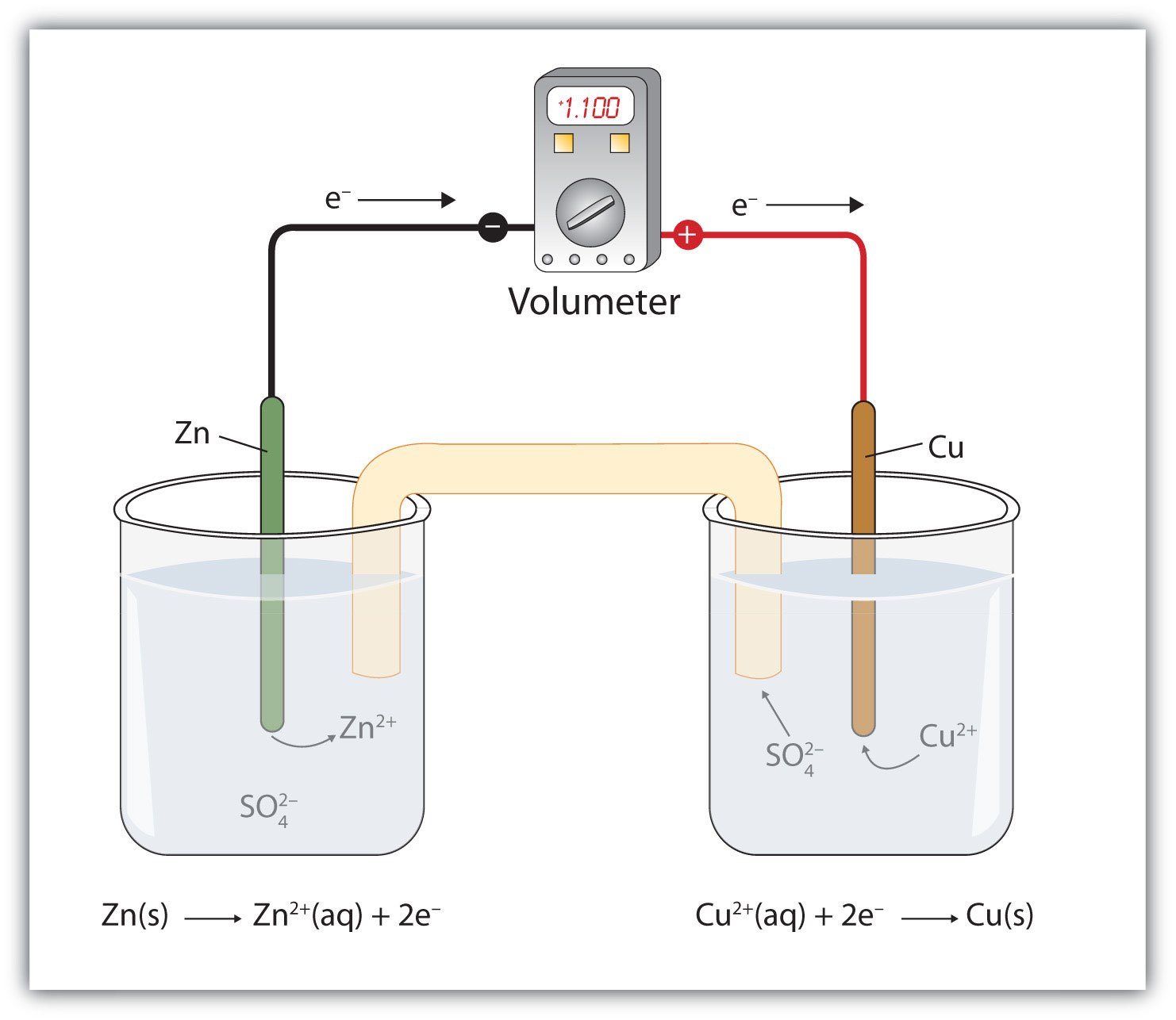One application of redox reactions requires that they be physically separated.

Even though the two half reactions are physically separated, a spontaneous redox reaction still occurs. However, in this case, the electrons transfer through the wire connecting the two half reactions; that is, this setup becomes a source of electricity. Useful work can be extracted from the electrons as they transfer from one side to the other—for example, a light bulb can be lit, or a motor can be operated. The apparatus as a whole, which allows useful electrical work to be extracted from a redox reaction, is called a voltaic (galvanic) cellAn apparatus that allows for useful electrical work to be extracted from a redox reaction..

Each individual system that contains a half reaction is called a half cellA part of a voltaic cell that contains one half reaction.. The half cell that contains the oxidation reaction is called the anodeThe half cell that contains the oxidation reaction., while the half cell that contains the reduction reaction is called the cathodeThe half cell that contains the reduction reaction.. The cathode and anode collectively are the electrodesThe cathode or anode of a voltaic cell. of the voltaic cell. Because electrons are coming from the anode, the anode is considered the negative electrode of the cell, while the cathode is considered the positive electrode of the cell. Finally, because electrons are moving from one half cell to the other, a charge imbalance builds up as the reaction proceeds. To counter that, a salt bridgeA part of a voltaic cell that contains a solution of some ionic compound whose ions migrate to either side of the voltaic cell to maintain the charge balance. is used; the salt bridge contains a solution of some ionic compound whose ions migrate to either side of the voltaic cell to maintain the charge balance.

The tendency for electrons to go from one half cell to another is called the voltageThe tendency for electrons to go from one half cell to another. of the voltaic cell, represented by E. Sometimes the term potential is used to represent the voltage of a cell. Voltage is expressed in volts (V). The voltage of a voltaic cell is determined by the difference in the tendencies of the individual half cells and is characteristic of a given redox reaction when concentrations are specific (1.0 M for dissolved species and 1.0 atm for gases). Because the voltage of a redox reaction is determined by the difference of the tendencies of the individual half reactions, absolute voltages are unnecessary; only relative voltages of each half reaction are needed. The relative voltage of each half cell is represented as E1/2 and is based on the standard that the E1/2 for the reaction

H+ + e → 1/2H2

is assigned to be exactly 0.000 V under standard conditions of pressure and concentration. Table 14.1 "Standard Reduction Potentials of Half Reactions" lists some relative E1/2 values for some half reactions. Note that all half reactions are listed as reduction reactions, so these values are called the standard reduction potentialsThe voltage of a reduction half reaction relative to the hydrogen half reaction. of each half reaction.

Table 14.1 Standard Reduction Potentials of Half Reactions

Reduction Half Reaction E1/2 (V)
F2 + 2e → 2F 2.87
Ce4+ + e → Ce3+ 1.61
MnO4 + 8H+ + 5e → Mn2+ + 4H2O 1.51
Cl2 + 2e → 2Cl 1.36
O2 + 4H+ + 4e → 2H2O 1.23
Br2 + 2e → 2Br 1.06
NO3 + 4H+ + 3e → NO + 2H2O 0.96
Ag+ + e → Ag 0.80
Fe3+ + e → Fe2+ 0.77
I2 + 2e → 2I 0.54
Cu2+ + 2e → Cu 0.34
AgCl + e → Ag + Cl 0.222
Sn4+ + 2e → Sn2+ 0.15
2H+ + 2e → H2 0.000
Pb2+ + 2e → Pb −0.126
Ni2+ + 2e → Ni −0.25
Cr3+ + e → Cr2+ −0.41
Fe2+ + 2e → Fe −0.44
Cr3+ + 3e → Cr −0.74
Zn2+ + 2e → Zn −0.76
Cr2+ + 2e → Cr −0.91
Ba2+ + 2e → Ba −1.57
Al3++ 3e → Al −1.66
Mg2+ + 2e → Mg −2.37
Na+ + e → Na −2.714
Li+ + e → Li −3.045

Table 14.1 "Standard Reduction Potentials of Half Reactions" lists only reduction reactions, but a redox reaction has a reduction and an oxidation. To make the oxidation reaction, simply reverse the reduction reaction in Table 14.1 "Standard Reduction Potentials of Half Reactions" and change the sign on the E1/2 value. If the reduction potential is negative, make the voltage for the oxidation positive; if the reduction potential is positive, make the voltage for the oxidation negative.

### Example 6

What is the value of E1/2 for this half reaction?

Ag + Cl → AgCl + e

Solution

The given reaction is the reverse of this reaction:

AgCl + e → Ag + Cl E1/2 = 0.222 V

Therefore, the E1/2 of the given reaction is −0.222 V.

Test Yourself

What is the value of E1/2 for this half reaction?

Na → Na+ + e

2.714 V

To determine the overall voltage of a particular voltaic cell, simply combine the voltages of the oxidation and reduction half reactions. Even if you need to take a multiple of a half reaction for the electrons to cancel, do not take the multiple of the E1/2. Use the values directly as is from Table 14.1 "Standard Reduction Potentials of Half Reactions".

Spontaneous redox reactions have positive overall voltages. If the voltage of the reaction as written is negative, it is not spontaneous in that direction. Rather, the reverse reaction is the spontaneous redox reaction.

### Example 7

What is the voltage of a voltaic cell based on this reaction? Is the reaction spontaneous as written?

2NO3 + 8H+ + 3Cu → 2NO + 4H2O + 3Cu2+

Solution

The overall redox reaction is formed from these two half reactions:

NO3 + 4H+ + 3e → NO + 2H2O E1/2 = 0.96 V Cu2+ + 2 e → Cu E1/2 = 0.34 V

The second reaction is reversed in the overall redox reaction, so its voltage changes sign from the reduction reaction:

Cu → Cu2+ + 2 e E1/2 = −0.34 V

To obtain the voltage of the voltaic cell based on the overall reaction, we simply combine the two voltages of the half reactions:

E = 0.96 + (−0.34) = 0.62 V

Because the overall voltage is positive, the reaction is spontaneous as written.

Test Yourself

What is the voltage of a voltaic cell based on this reaction? Is the reaction spontaneous as written?

5Ni + 2MnO4 + 16H+ → 3Mn2+ + 8H2O + 5Ni2+

1.76 V; spontaneous

Technically, any redox reaction can be set up to make a voltaic cell. In modern society, however, only certain redox reactions are put to practical use. A portable voltaic cell that generates electricity to power devices for our convenience is called a batteryA portable voltaic cell that generates electricity to power devices for our convenience.. All batteries are based on redox reactions.

The first battery (called a “voltaic pile”) was constructed by the Italian scientist Alessandro Volta in 1800 and was based on the copper/zinc reaction depicted in Figure 14.1 "A Redox Reaction in Which the Two Half Reactions Are Physically Separated". Unfortunately, it was messy, requiring quantities of copper and zinc salts dissolved in water. In 1866, the French scientist Georges Leclanché invented the dry cellA modern battery that does not contain large amounts of aqueous solution., a precursor to today’s modern battery. A schematic of a dry cell is shown in Figure 14.2 "Dry Cells". The zinc case and the central carbon rod serve as the anode and cathode, respectively. The other reactants are combined into a moist paste that minimizes free liquid, so the battery is less messy (hence the name dry cell). The actual redox reaction is complex but can be represented by the following redox reaction:

Zn + 2MnO2 + 2NH4+ → Zn2+ + Mn2O3 + 2NH3 + H2O

A dry cell has a voltage of about 1.56 V. While common and useful, dry cells have relatively short lifetimes and contain acidic components. They also cannot be recharged, so they are one-use only. Batteries that can be used only once are called primary batteriesA battery that cannot be recharged..

Figure 14.2 Dry Cells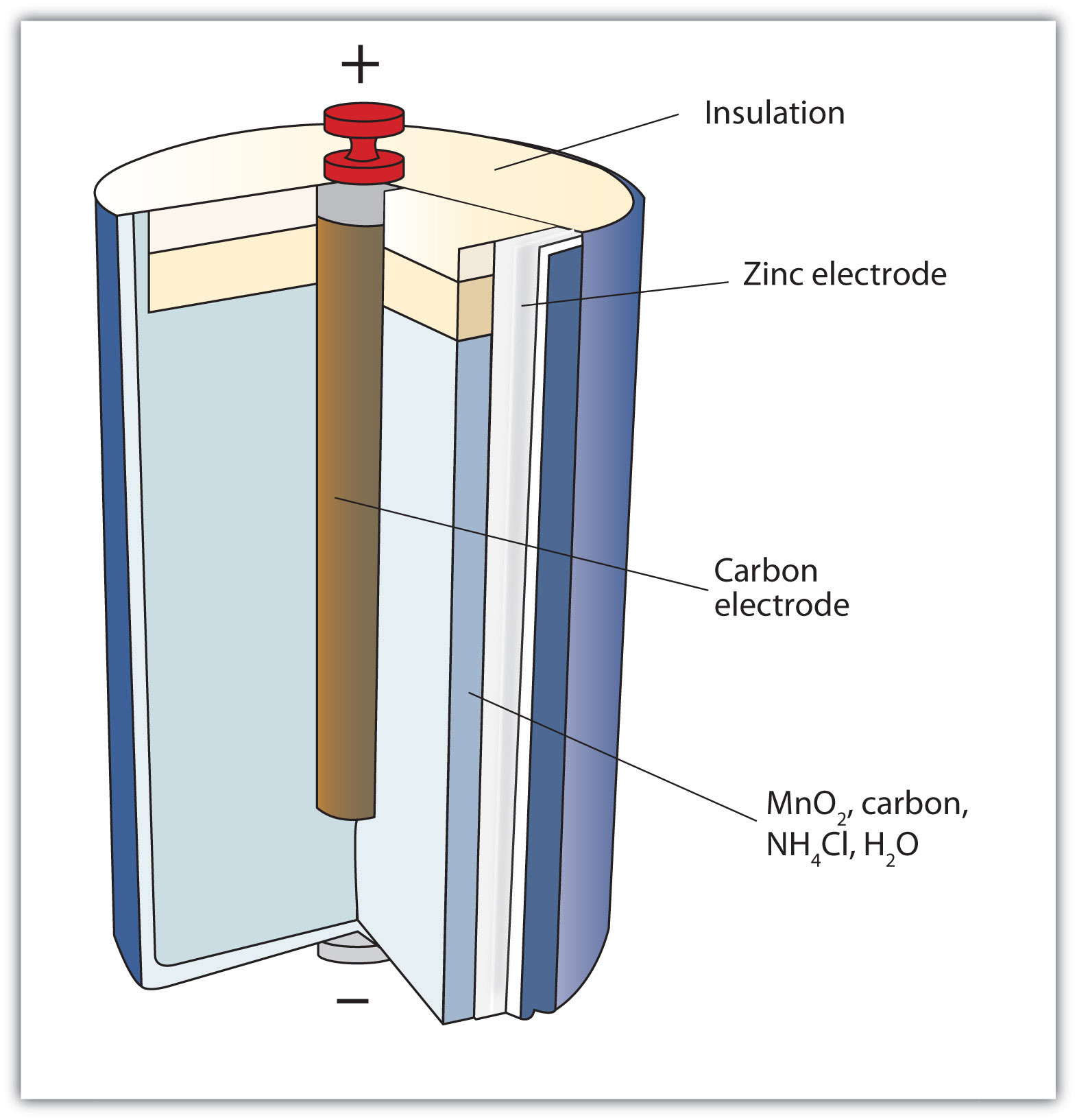The Leclanché dry cell is a common type of battery.

In the late 1950s, Lewis Urry of the Eveready Battery Company in Ohio invented the alkaline batteryA type of dry cell that contains an alkaline (i.e., basic) moist paste, rather than an acidic paste. (still marketed today under the trade name Energizer). Alkaline batteries are similar to dry cells, but they use a basic moist paste rather than an acidic one. Moreover, the net amount of base does not change during the course of the redox reaction. The overall redox reaction is as follows:

Zn + 2MnO2 → ZnO + Mn2O3

Alkaline batteries have the advantage of being longer lasting and holding their voltage better—about 1.54 V—throughout their lifetime.

A common type of battery, especially with the increased popularity of personal electronic devices, is the button battery (Figure 14.3 "Button Batteries"). A button battery is a small battery that can power small electronic devices; the batteries can be as small as 5 mm across. Two popular redox reactions used for button batteries are the alkaline dry-cell reaction and a silver oxide-based reaction:

Zn + Ag2O → ZnO + 2Ag

Some button batteries use a lithium-based redox reaction, typified by this anode reaction:

Li → Li+ + e E1/2 = 3.045 V

The actual redox reaction depends on the composition of the cathode and is variable depending on voltage. Lithium batteries can also be used for applications that require more energy, such as portable computers and electric vehicles. Some lithium-based batteries are rechargeable and can be used over and over again; such batteries are called secondary batteriesA battery that can be recharged..

Figure 14.3 Button Batteries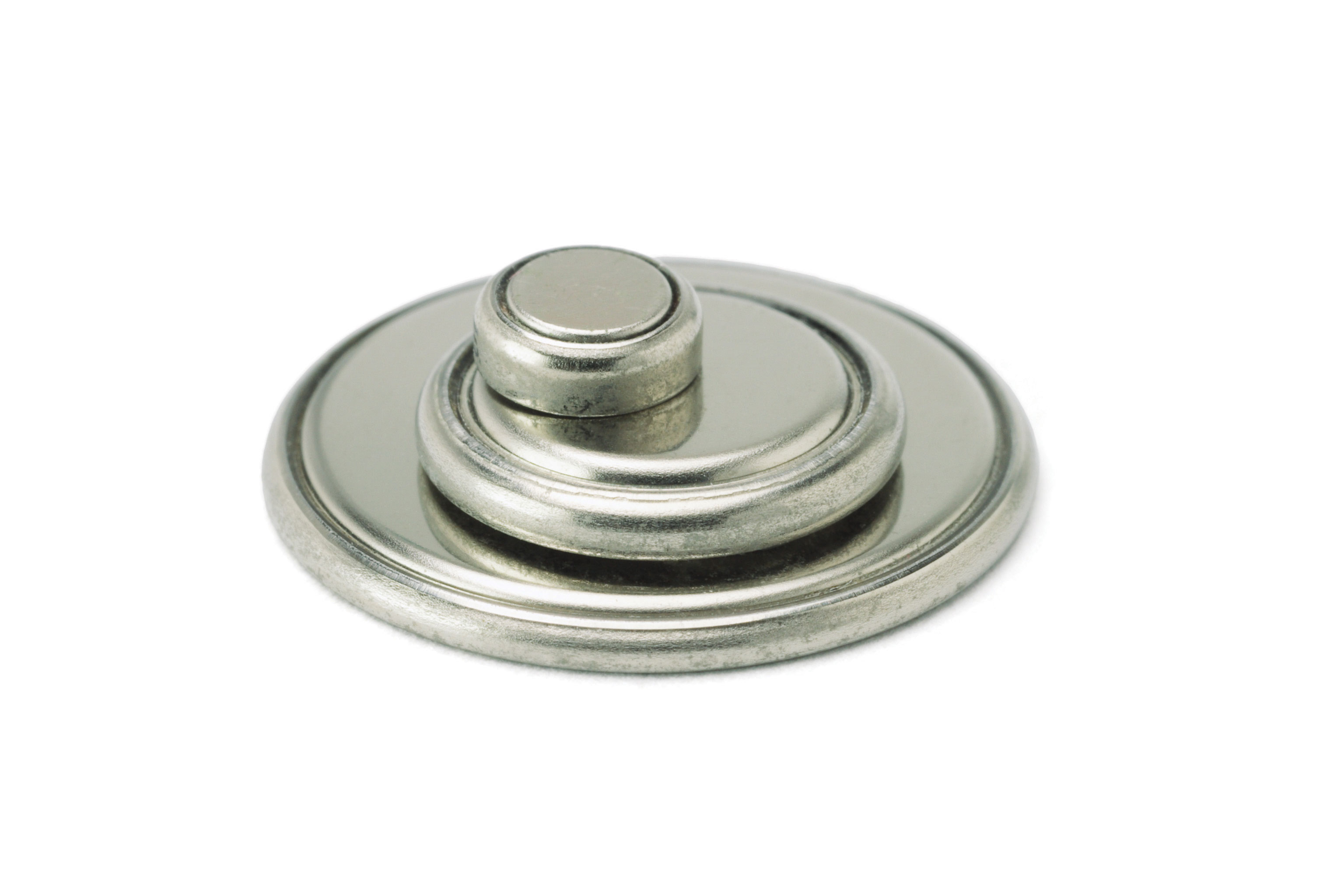Button batteries like those seen here can be used for a variety of portable electronics, from watches and hearing aids to handheld gaming devices.

An important secondary battery is the lead storage battery, shown in Figure 14.4 "Lead Storage Batteries". The lead storage battery is based on this redox reaction:

Pb + PbO2 + 4H+ + SO42− → 2PbSO4 + 2H2O

The redox reaction produces about 2 V, but it is typical to tie several individual batteries together to generate a larger voltage. The lead storage battery has the distinction that the product of both half reactions is PbSO4, which as a solid accumulates on the many plates within each cell (Figure 14.4 "Lead Storage Batteries"). The lead storage battery is a secondary battery, as it can be recharged and reused many times. Because it is based on lead, these batteries are rather heavy. They should also be recycled when replaced so that potentially dangerous lead does not escape into the environment. Because of their characteristics, lead storage batteries are used to start large engines in automobiles, boats, and airplanes.

Figure 14.4 Lead Storage Batteries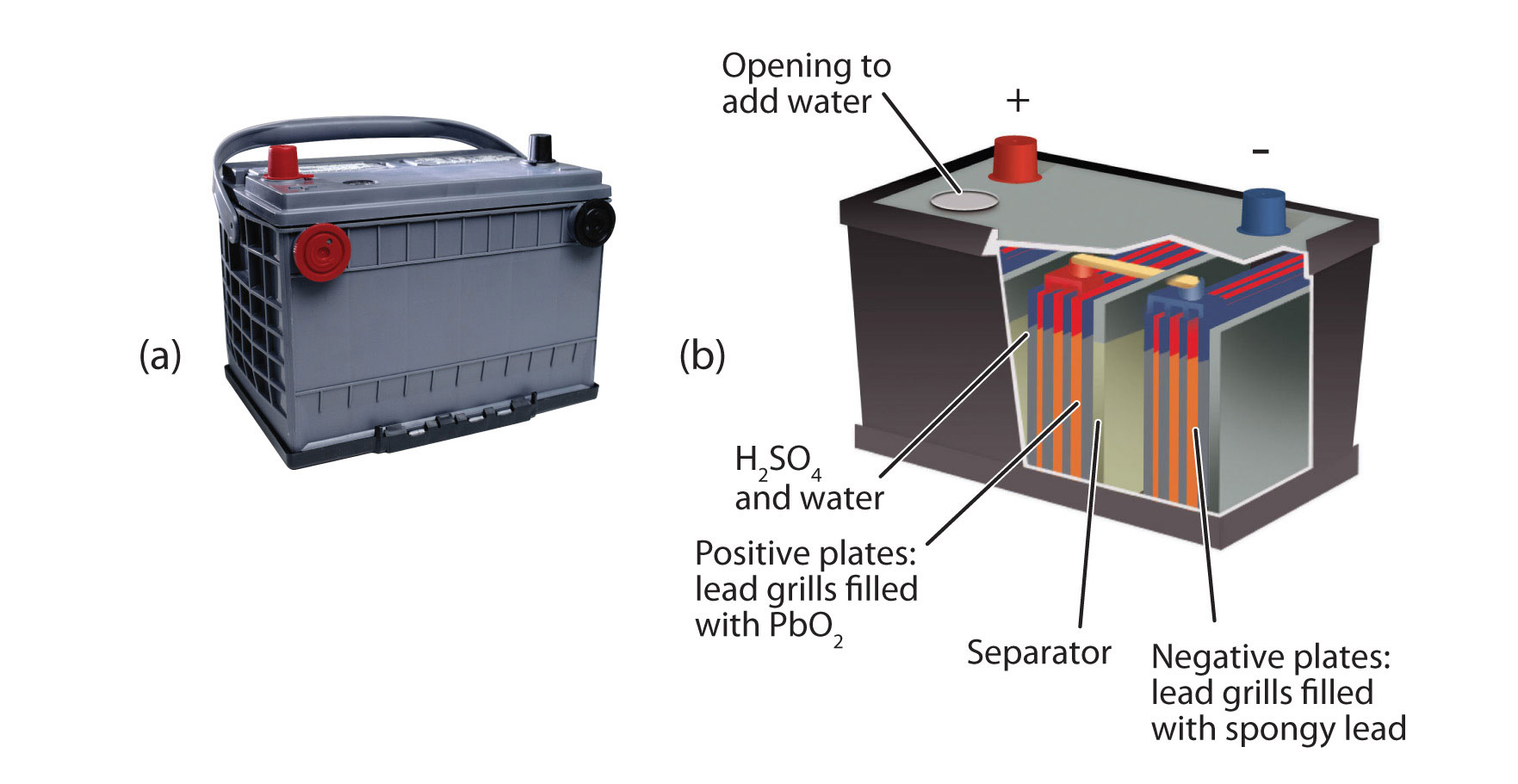(a) A photo of a lead storage battery. (b) A schematic diagram of a lead storage battery.

### Chemistry Is Everywhere: Fuel Cells

A fuel cell is a type of battery in which reactants flow continuously into a specialized reaction chamber, and products flow out continuously while electrons are extracted from the reaction. Because all reactions in a fuel cell consist of a fuel and an oxidizer undergoing a redox reaction, an introduction of fuel cells is at home in a discussion of redox chemistry.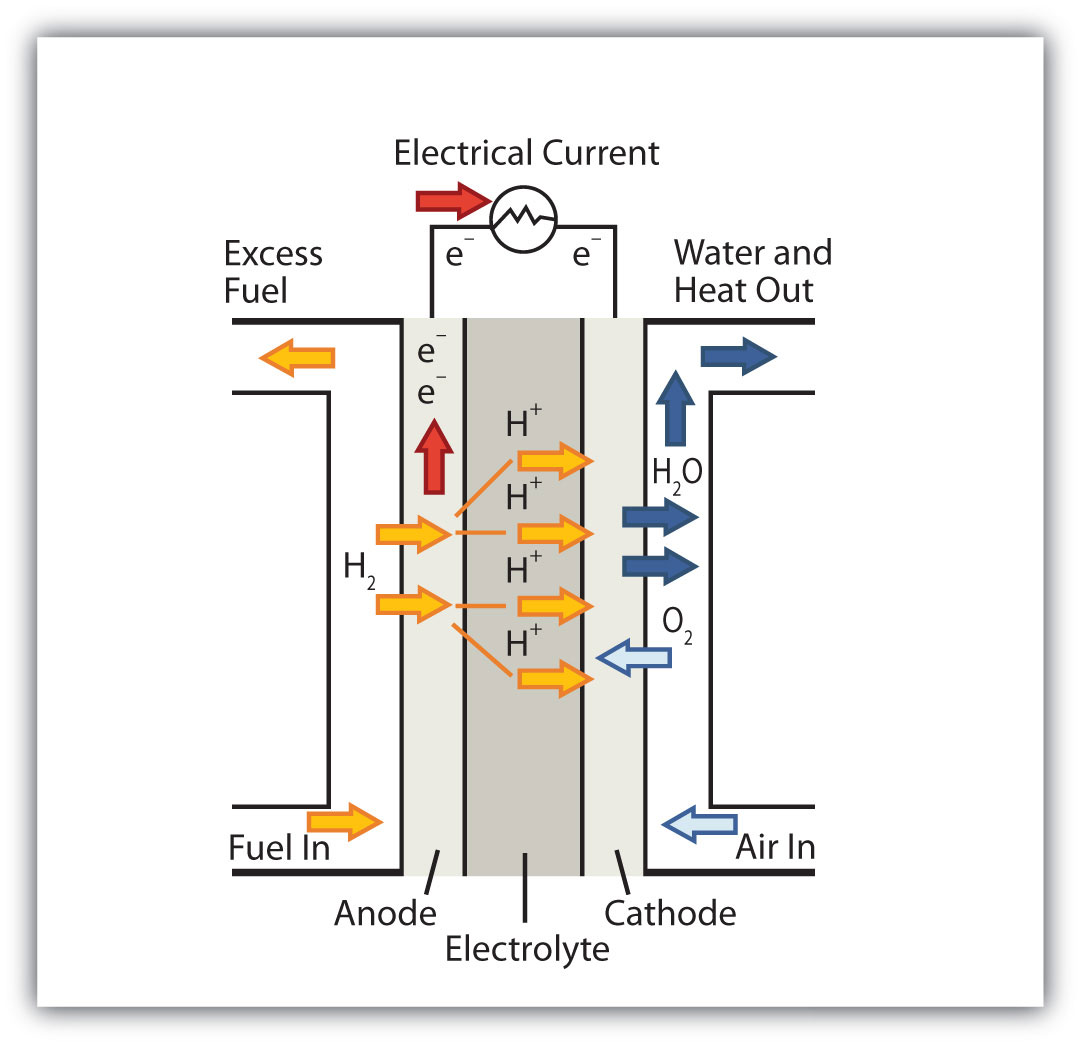This fuel cell uses H2 as the fuel and O2 as the oxidizer.

By far the most common fuel cell reaction is based on hydrogen and oxygen:

2H2 + O2 → 2H2O E = 1.23 V under standard conditions

However, fuel cells typically do not work under standard nor even optimal conditions, so they typically generate about 0.6–0.7 V. In this fuel cell, the only two products are water and electricity, so the fuel cell not only does not create pollution but also makes a by-product that in some environments is a valuable commodity (water). Other fuels can be used besides hydrogen; fuel cells have been developed that work on methane, methyl alcohol, ethyl alcohol, carbon-rich materials, and even magnesium metal.

Hydrogen-based fuel cells were and are used to provide electricity for manned space vehicles, partly because their only chemical product is water, which could be used for drinking. However, there has been a recent resurgence in interest in fuel cells because of their potential use in electric cars. Most electric cars run on conventional batteries, which can be very heavy and expensive to replace. It is thought that fuel cells, rather than conventional batteries, might be better sources of electricity for automobiles.

Several current barriers to fuel cell use in electric cars include capacity, cost, and overall energy efficiency. The 2008 Honda FCX, the first production model of a vehicle powered with a fuel cell, can hold 4.1 kg (just under 9 lb) of highly pressured H2 gas and has a range of 450 km (280 mi). It costs about $120,000–$140,000 to build, making the vehicle beyond the ability of most people to own. Finally, it always requires more energy to produce elemental hydrogen as a fuel than can be extracted from hydrogen as a fuel. As such, hydrogen is described as an energy carrier (like electricity) rather than an energy source (like oil and gas). This distinction points out a fundamental argument against fuel cells as a “better” power source.The 2008 Honda FCX was the first production car to use a fuel cell as a power source. Nonetheless, the car is in very limited service because of its need for relatively large quantities of elemental hydrogen as fuel.

The limitations notwithstanding, there is a lot of interest in fuel cell research. If ways can be found to circumvent their current limitations, fuel cells may become more and more common as power sources.

### Key Takeaways

• A voltaic cell produces electricity as a redox reaction occurs.
• The voltage of a voltaic cell can be determined by the reduction potentials of the half reactions.
• Voltaic cells are fashioned into batteries, which are a convenient source of electricity.

### Exercises

1. Draw the voltaic cell represented by this reaction and label the cathode, the anode, the salt bridge, the oxidation half cell, the reduction half cell, the positive electrode, and the negative electrode. Use Figure 14.1 "A Redox Reaction in Which the Two Half Reactions Are Physically Separated" as a guide.

Zn + 2Ag+ → Zn2+ + 2Ag
2. Draw the voltaic cell represented by this reaction and label the cathode, the anode, the salt bridge, the oxidation half cell, the reduction half cell, the positive electrode, and the negative electrode. Use Figure 14.1 "A Redox Reaction in Which the Two Half Reactions Are Physically Separated" as a guide.

3Mg + 2Cr3+ → 3Mg2+ + 2Cr
3. What is the voltage of this half reaction?

2F → F2 + 2e
4. What is the voltage of this half reaction?

Na → Na+ + e
5. What is the voltage of the voltaic cell in Exercise 1? Consult Table 14.1 "Standard Reduction Potentials of Half Reactions" for data.

6. What is the voltage of the voltaic cell in Exercise 2? Consult Table 14.1 "Standard Reduction Potentials of Half Reactions" for data.

7. Balance this redox reaction and determine its voltage. Is it spontaneous?

Li+ + Al → Li + Al3+
8. Balance this redox reaction and determine its voltage. Is it spontaneous?

Pb2+ + Ni → Pb + Ni2+
9. Balance this redox reaction and determine its voltage. Is it spontaneous?

Cu2+ + Ag + Cl → Cu + AgCl
10. Balance this redox reaction and determine its voltage. Is it spontaneous?

Mn2+ + Br2 → MnO4 + Br
11. Which reaction represents the cathode reaction in Exercise 7? The anode reaction?

12. Which reaction represents the cathode reaction in Exercise 8? The anode reaction?

13. Which reaction represents the cathode reaction in Exercise 9? The anode reaction?

14. Which reaction represents the cathode reaction in Exercise 10? The anode reaction?

15. A voltaic cell is based on this reaction:

Ni + 2Au+ → Ni2+ + 2Au

If the voltage of the cell is 0.33 V, what is the standard reduction potential of the Au+ + e → Au half reaction?

16. A voltaic cell is based on this reaction:

3Pb + 2V3+ → 3Pb2+ + 2V

If the voltage of the cell is −0.72 V, what is the standard reduction potential of the V3+ + 3e → V half reaction?

17. What species is being oxidized and what species is being reduced in a dry cell?

18. What species is being oxidized and what species is being reduced in an alkaline battery?

19. What species is being oxidized and what species is being reduced in a silver oxide button battery?

20. What species is being oxidized and what species is being reduced in a lead storage battery?

21. Based on the data in Table 14.1 "Standard Reduction Potentials of Half Reactions", what is the highest voltage battery you can construct?

22. Based on the data in Table 14.1 "Standard Reduction Potentials of Half Reactions", what is the lowest voltage battery you can construct? (This may be more challenging to answer than Exercise 21.)

1.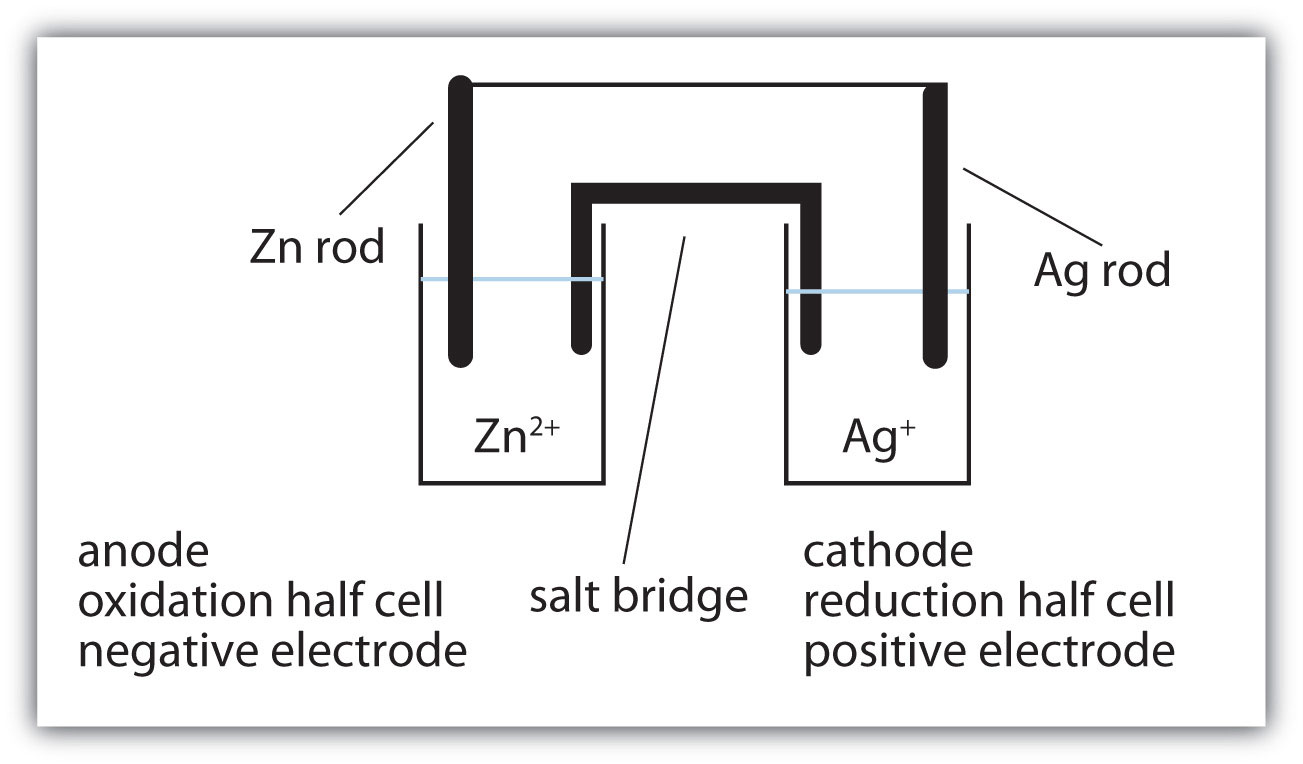2. −2.87 V

3. 1.56 V

4. 3Li+ + Al → 3Li + Al3+; −1.39 V; not spontaneous

5. Cu2+ + 2Ag + 2Cl → Cu + 2AgCl; 0.12 V; spontaneous

6. cathode reaction: Li+ + e → Li; anode reaction: Al → Al3+ + 3e

7. cathode reaction: Cu2+ + 2e → Cu; anode reaction: Ag + Cl → AgCl + e

8. 0.08 V

9. oxidized: Zn; reduced: Mn

10. oxidized: Zn; reduced: Ag

11. 5.92 V from the reaction of F2 and Li

## 14.4 Electrolysis

### Learning Objectives

1. Describe electrolysis from a perspective of redox reactions.
2. Give examples of electrolysis applications.

Up to this point, we have considered redox reactions for processes that are spontaneous. When set up as a voltaic cell or battery, such reactions can be used as a source of electricity. However, it is possible to go in the other direction. By forcing electricity into a cell, we can make a redox reaction occur that normally would not be spontaneous. Under these circumstances, the cell is called an electrolytic cellA cell into which electricity is forced to make a nonspontaneous reaction occur., and the process that occurs in the cell is called electrolysisThe process of making a nonspontaneous redox reaction occur by forcing electricity into a cell. (Figure 14.5 "Electrolysis").

Figure 14.5 Electrolysis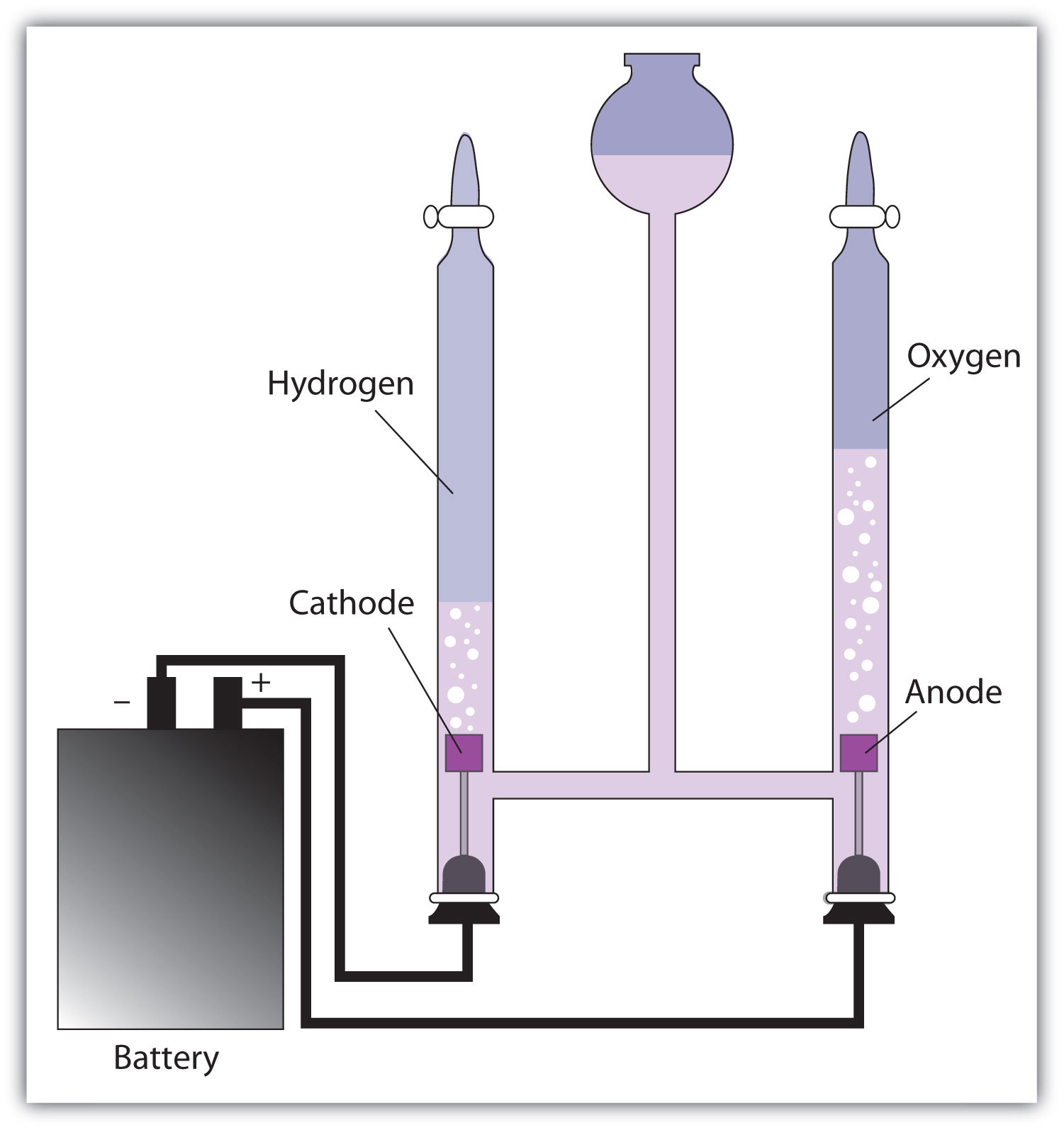In an electrolytic cell, electricity is forced through the cell to induce a nonspontaneous redox reaction. Here, the redox reaction 2H2O → 2H2 + O2 is being caused by the introduction of electricity, which is supplied by the battery.

Electrolysis has many applications. For example, if NaCl is melted at about 800°C in an electrolytic cell and an electric current is passed through it, elemental sodium will appear at the cathode and elemental chlorine will appear at the anode as the following two reactions occur:

Na+ + e → Na 2Cl → Cl2 + 2e

Normally we expect elemental sodium and chlorine to react spontaneously to make NaCl. However, by using an input of electricity, we can force the opposite reaction to occur and generate the elements. Lithium, potassium, and magnesium can also be isolated from compounds by electrolysis.

Another element that is isolated by electrolysis is aluminum. Aluminum formerly was a difficult metal to isolate in its elemental form; in fact, the top of the Washington Monument has a 2.8 kg cap of aluminum metal, which at the time—1884—was the largest piece of elemental aluminum ever isolated. However, in 1886 the American Charles Hall and the Frenchman Paul Héroult almost simultaneously worked out an electrolytic process for isolating aluminum from bauxite, an ore of aluminum whose chemical formula is AlOx(OH)3 − 2x. The basic reactions are as follows:

Al3+ + 3e → Al 2O2− → O2 + 4e

With the development of the Hall-Héroult process, the price of aluminum dropped by a factor of over 200, and aluminum metal became common. So much elemental aluminum is produced in the United States each year that it has been estimated that the electrolysis of aluminum uses 5% of all the electricity in the country. (Recycling aluminum requires about 1/70th the energy of refining aluminum from ore, which illustrates the tremendous energy savings that recycling provides.)

Another application of electrolysis is electroplatingThe deposition of a thin layer of metal on an object for protective or decorative purposes., which is the deposition of a thin layer of metal on an object for protective or decorative purposes (Figure 14.6). Essentially, a metal object is connected to the cathode of an electrolytic cell and immersed in a solution of a particular metal cation. When the electrolytic cell is operated, a thin coating of the metal cation is reduced to the elemental metal on the surface of the object; the thickness of the coating can be as little as a few micrometers (10−6 m). Jewelry, eating utensils, electrical contacts, and car parts like bumpers are common items that are electroplated. Gold, silver, nickel, copper, and chromium are common metals used in electroplating.

Figure 14.6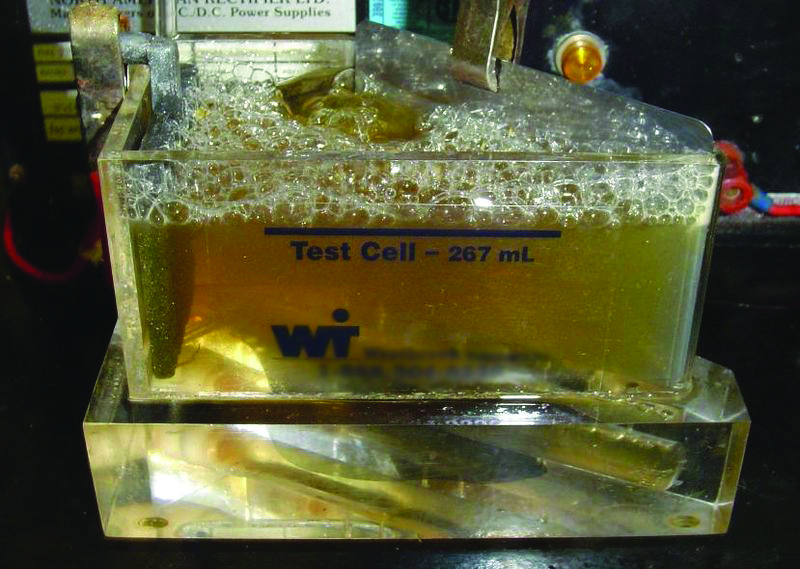### Key Takeaways

• Electrolysis is the forcing of a nonspontaneous redox reaction to occur by the introduction of electricity into a cell from an outside source.
• Electrolysis is used to isolate elements and electroplate objects.

### Exercises

1. Define electrolytic cell.

2. How does the operation of an electrolytic cell differ from a voltaic cell?

3. List at least three elements that are produced by electrolysis.

4. Write the half reactions for the electrolysis of the elements listed in Exercise 3.

5. Based on Table 14.1 "Standard Reduction Potentials of Half Reactions", what voltage must be applied to an electrolytic cell to electroplate copper from Cu2+?

6. Based on Table 14.1 "Standard Reduction Potentials of Half Reactions", what voltage must be applied to an electrolytic cell to electroplate aluminum from Al3+?

1. an electrochemical cell in which charge is forced through and a nonspontaneous reaction occurs

2. any three of the following: Al, K, Li, Na, Cl2, or Mg

3. 0.34 V

## 14.5 End-of-Chapter Material

1. Oxidation was once defined as chemically adding oxygen to a substance. Use this reaction to argue that this definition is consistent with the modern definition of oxidation.

2Mg + O2 → 2MgO
2. Reduction was once defined as chemically adding hydrogen to a substance. Use this reaction to argue that this definition is consistent with the modern definition of reduction.

C2H2 + 2H2 → C2H6
3. Assign oxidation numbers to the atoms in each substance.

1. Kr (krypton)
2. krypton tetrafluoride (KrF4)
3. dioxygen difluoride (O2F2)
4. Assign oxidation numbers to the atoms in each substance.

1. lithium hydride (LiH)
2. potassium peroxide (K2O2)
3. potassium fluoride (KF)
5. N atoms can have a wide range of oxidation numbers. Assign oxidation numbers for the N atom in each compound, all of which are known compounds.

1. N2O5
2. N2O4
3. NO2
4. NO
5. N2H4
6. NH3
6. Cr atoms can have a wide range of oxidation numbers. Assign oxidation numbers for the Cr atom in each compound, all of which are known compounds.

1. Na2CrO4
2. Na2Cr2O7
3. CrF5
4. CrCl3
5. CrCl2
7. Balance this redox reaction by inspection.

S8 + O2 → SO2
8. Balance this redox reaction by inspection.

C18H38 + O2 → CO2 + H2O
9. Balance this redox reaction by the half reaction method by assuming an acidic solution.

Cr2O72− + Fe → Cr3+ + Fe3+
10. Balance the redox reaction in Exercise 9 by the half reaction method by assuming a basic solution.

11. The uranyl ion (UO22+) is a fairly stable ion of uranium that requires strong reducers to reduce the oxidation number of uranium further. Balance this redox reaction using the half reaction method by assuming an acidic solution.

UO22+ + HN3 → U + N2
12. Balance the redox reaction in Exercise 11 by the half reaction method by assuming a basic solution.

13. Zinc metal can be dissolved by acid, which contains H+ ions. Demonstrate that this is consistent with the fact that this reaction has a spontaneous voltage:

Zn + 2H+ → Zn2+ + H2
14. Copper metal cannot be dissolved by acid, which contains H+ ions. Demonstrate that this is consistent with the fact that this reaction has a nonspontaneous voltage:

Cu + 2H+ → Cu2+ + H2
15. A disproportionation reaction occurs when a single reactant is both oxidized and reduced. Balance and determine the voltage of this disproportionation reaction. Use the data in Table 14.1 "Standard Reduction Potentials of Half Reactions".

Cr2+ → Cr + Cr3+
16. A disproportionation reaction occurs when a single reactant is both oxidized and reduced. Balance and determine the voltage of this disproportionation reaction. Use the data in Table 14.1 "Standard Reduction Potentials of Half Reactions".

Fe2+ → Fe + Fe3+
17. What would be overall reaction for a fuel cell that uses CH4 as the fuel?

18. What would be overall reaction for a fuel cell that uses gasoline (general formula C8H18) as the fuel?

19. When NaCl undergoes electrolysis, sodium appears at the cathode. Is the definition of cathode the same for an electrolytic cell as it is for a voltaic cell?

20. When NaCl undergoes electrolysis, chlorine appears at the anode. Is the definition of anode the same for an electrolytic cell as it is for a voltaic cell?

21. An award is being plated with pure gold before it is presented to a recipient. If the area of the award is 55.0 cm2 and will be plated with 3.00 µm of Au, what mass of Au will be plated on the award? The density of Au is 19.3 g/cm3.

22. The unit of electrical charge is called the coulomb (C). It takes 96,500 coulombs of charge to reduce 27.0 g of Al from Al3+ to Al metal. At 1,040 cm3, how many coulombs of charge were needed to reduce the aluminum in the cap of the Washington monument, assuming the cap is pure Al? The density of Al is 2.70 g/cm3.

1. As oxygen is added to magnesium, it is being oxidized. In modern terms, the Mg atoms are losing electrons and being oxidized, while the electrons are going to the O atoms.

1. Kr: 0
2. Kr: +4; F: −1
3. O: +1; F: −1
1. +5
2. +4
3. +4
4. +2
5. −2
6. −3
2. S8 + 8O2 → 8SO2

3. 14H+ + Cr2O72− + 2Fe → 2Cr3+ + 7H2O + 2Fe3+

4. 6HN3 + UO22+ → U + 2H2O + 9N2 + 2H+

5. The voltage of the reaction is +0.76 V, which implies a spontaneous reaction.

6. 3Cr2+ → Cr + 2Cr3+; −0.50 V

7. CH4 + 2O2 → CO2 + 2H2O

8. yes because reduction occurs at the cathode

9. 0.318 g